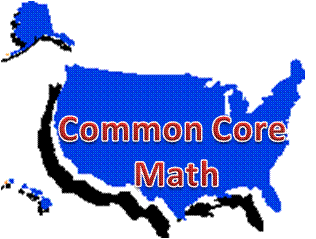# Common Core High School Number and Quantity Teaching and Learning Resources

## Domain: RN: The Real Number System• RN-A: Extend the properties of exponents to rational exponents

• RN-B: Use properties of rational and irrational numbers### RN-A: Extend the properties of exponents to rational exponents

Standards:

• RN-A.1. Explain how the definition of the meaning of rational exponents follows from extending the properties of integer exponents to those values, allowing for a notation for radicals in terms of rational exponents.  For example, we define 51/3 to be the cube root of 5 because we want(51/3)3 = 5(1/3)3 to hold, so (51/3)3 must equal 5.
• RN-A.2. Rewrite expressions involving radicals and rational exponents using the properties of exponents.

Technology-enhanced investigations:

Mathwords.com: Math Dictionary: Key vocabulary for this domain.  Use with RN-A, RN-B:

 exponent rules irratiional numbers radical rules rational numbers integers radical rational exponents real numbers

Interactive Mathematics: Exponents and Radicals begins with introducing laws of exponents and simplifying expressions with integral exponents, then delves into fractional exponents, simplest radical form, addition and subtraction of radicals, multiplication and division of radicals, and equations with radicals.  Use with RN-A.1 and RN-A.2.

Study.com: Algebra:  Each of the following chapters includes video lessons with sample problems and a short quiz in each video.  Multiple choice chapter exams are included.

LearnZillion:

• Lesson set: Relate rational exponents to integer exponents, use radical notation: Five video lessons: Apply exponents to physical models, relate fractional exponents to integer exponents, solve for variables with rational exponents; perform operations and solve equations with rational exponents.  Aligns with RN-A.1.
• Lesson set: Rewrite expressions involving radicals and rational exponents: Five video lessons: Simplify square roots using physical models, simplify radical expressions by factoring, estimate the value of square roots, write equivalent radical and rational exponent expressions, explore volume using radical and rational exponent expressions.  Aligns with RN-A.2.

Ohio Resource Center on YouTube: Tutorials for High School Mathematics: Scientific Notation: Basics for writing numbers in scientific notation.

Purple Math: Fractional (Rational) Exponents: Basic theory

West Texas A & M University: Virtual Math Lab:

• College Algebra: Tutorial 5: Rational Exponents: After completing this tutorial, learners should be able to: 1. Rewrite a rational exponent in radical notation. 2. Simplify an expression that contains a rational exponent. 3. Use rational exponents to simplify a radical expression. Video demonstrations and practice problems are included in above.
• Intermediate Algebra: Tutorial 38: Rational Exponents: After completing this tutorial, learners should be able to: 1. Rewrite a rational exponent in radical notation. 2. Simplify an expression that contains a rational exponent. 3. Use rational exponents to simplify a radical expression. Practice problems are included in above.

Multiple Choice:

Khan Academy: Practice questions with videos.

MathBitsNotebook: Algebra 1: Exponents includes lessons, then practice problems on integer exponent skills (numeric and algebraic) and rational fraction exponent skills.  Use with RN-A.1 and RN-A.2.

Constructed-response:

AlgebraLab.org: Scientific Notation Introduction and fill-in the blank practice exercises converting to scientific notation and decimal notation from the scientific form.

Edinformatics.com: Scientific Notation Problem Generator.  Randomly generated problems in either scientific notation or standard notation representation of a number will be shown. Includes multiplication, division, and word problems involving scientific notation with positive and negative exponents.  Fill in the blanks, then check answer.

Hot Math: Rational Exponents: Practice problems with rational exponents.  Hints and solution steps provided.

Khan Academy: Practice questions with videos.

Math Warehouse: Simplify fraction exponents: Formula and examples of how to simplify fraction exponents, including practice problems for learners.

University of Hawaii: Math 110 College Algebra notes: Chapter P.3: Rational Exponents and Radicals: Within this lesson with worked examples are multiple exercises for learners to complete (paper-pencil needed) on simplifying expressions with rational exponents and radicals.

Zweigmedia.com:  Algebra Review Tutorials and examples using fill-in or multiple choice to test understanding.  Learners can also choose to do game versions of some topics.  Use with RN-A:

Illustrative Mathematics: Number and Quantity:

Mathematics Vision Project, Secondary 2 Student Edition:

• Module 3: Quadratic Equations: This module contains 10 classroom tasks.  Real number standards addressed in Module 3 include  RN-A.1, RN-A.2 in tasks 1-4 and RN-B.3 within tasks 9-10.

### RN-B: Use properties of rational and irrational numbers

Standards:

• RN-B.3. Explain why the sum or product of two rational numbers is rational; that the sum of a rational number and an irrational number is irrational; and that the product of a nonzero rational number and an irrational number is irrational.

Technology-enhanced investigations:

Khan Academy: Algebra 1: Irrational Numbers includes video proofs on sums and products of rational and irrational numbers that align with RN-B.3.

LearnZillion: Lesson set: Identify rules for adding and multiplying rational and irrational numbers: Four video lessons: Distinguish between rational and irrational numbers, predict the result of adding and subtracting rational and irrational numbers, multiply rational and irrational numbers, rationalize the denominator.  Aligns with RN-B.3.

Mathematics Assessment Project, CLASSROOM CHALLENGES, Formative Assessment Lessons: Task 424: Classifying Rational and Irrational Numbers and Task 434: Evaluating Statements about Rational and Irrational Numbers.  These lessons relate to the standard RN-B.3: Use rational and irrational numbers.

Multiple Choice:

Khan Academy: Practice questions with videos.

MathBitsNotebook: Algebra 1: Rationals and Irrationals includes lessons on rationals and irrationals, sum/product of two rationals, two irrationals, rationals and irrationals, then practice problems on rational/irrational values, and a speedometer quiz game on these concepts.  Use with RN-B.3.

Shmoop: MATH.N-RN.3 includes a quiz with sums and products involving radicals and problems illustrating RN-B.3.

Constructed-response:

JMAP.org: Regents Exam Questions N.RN.B.3: Operations with Radicals 1 (pdf) combines multiple choice and constructed response questions.  Aligns with RN-B.3.

Illustrative Mathematics: Number and Quantity:

Mathematics Assessment Project: Standards: High School: Number and Quantity: Quantities:

Mathematics Vision Project, Secondary 2 Student Edition:

• Module 3: Quadratic Equations: This module contains 10 classroom tasks.  Real number standards addressed in Module 3 include  RN-A.1, RN-A.2 in tasks 1-4 and RN-B.3 within tasks 9-10.